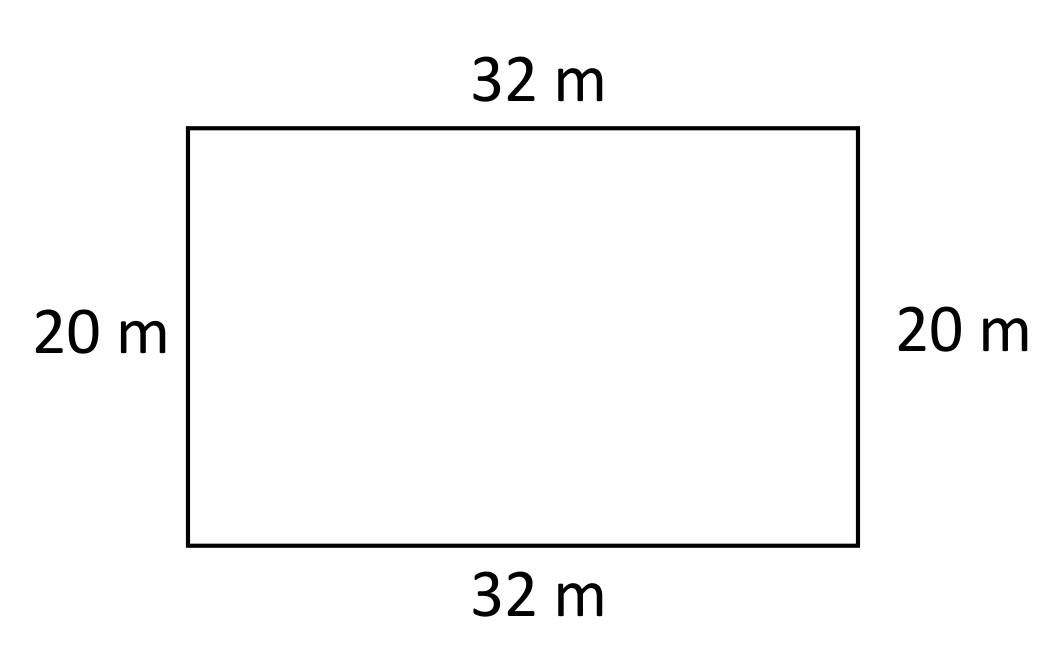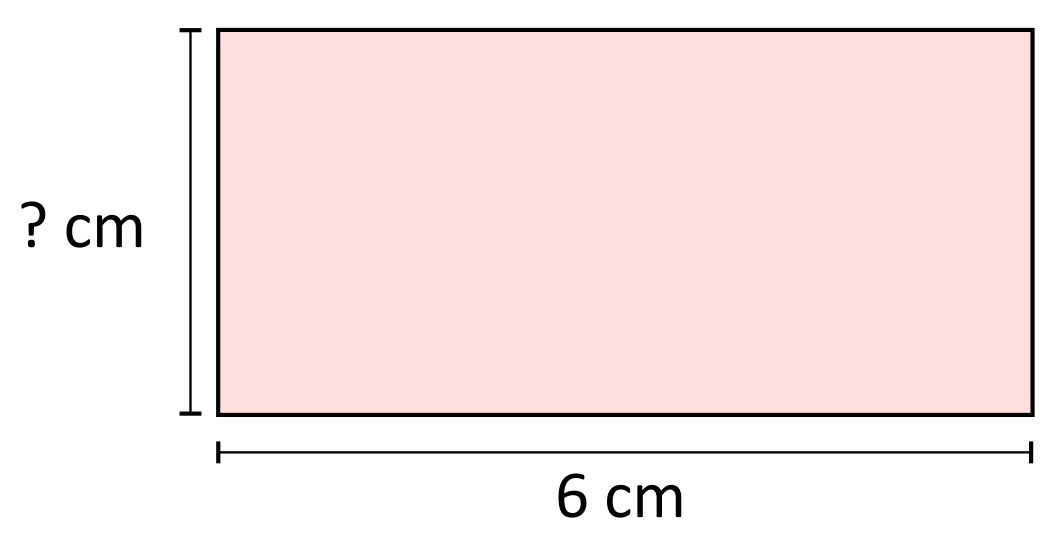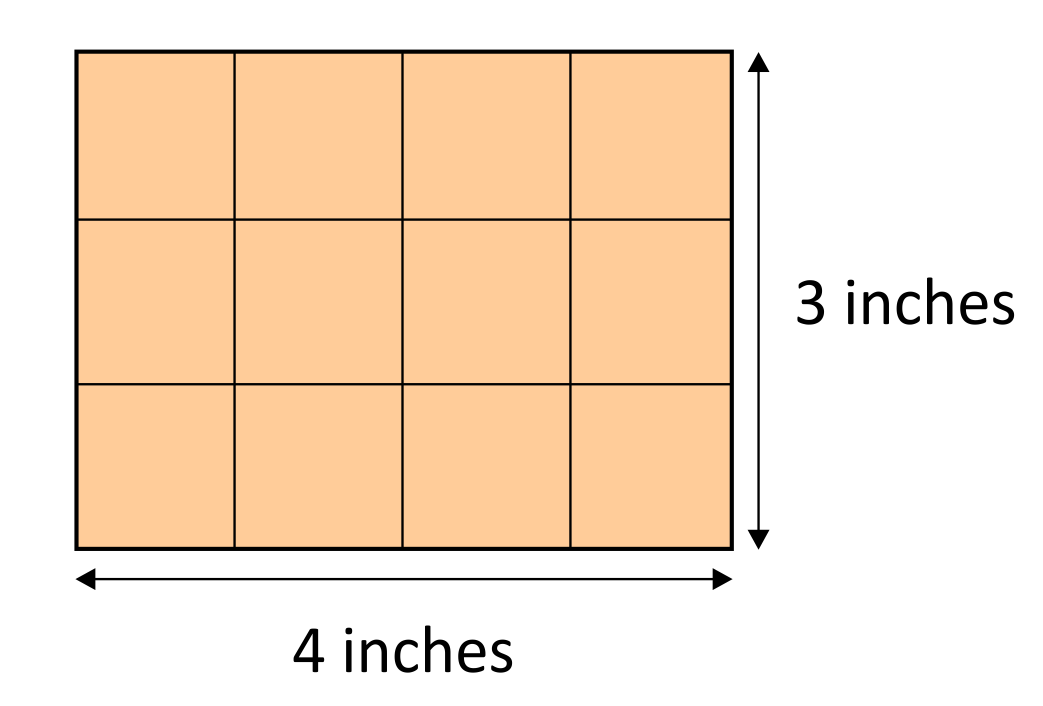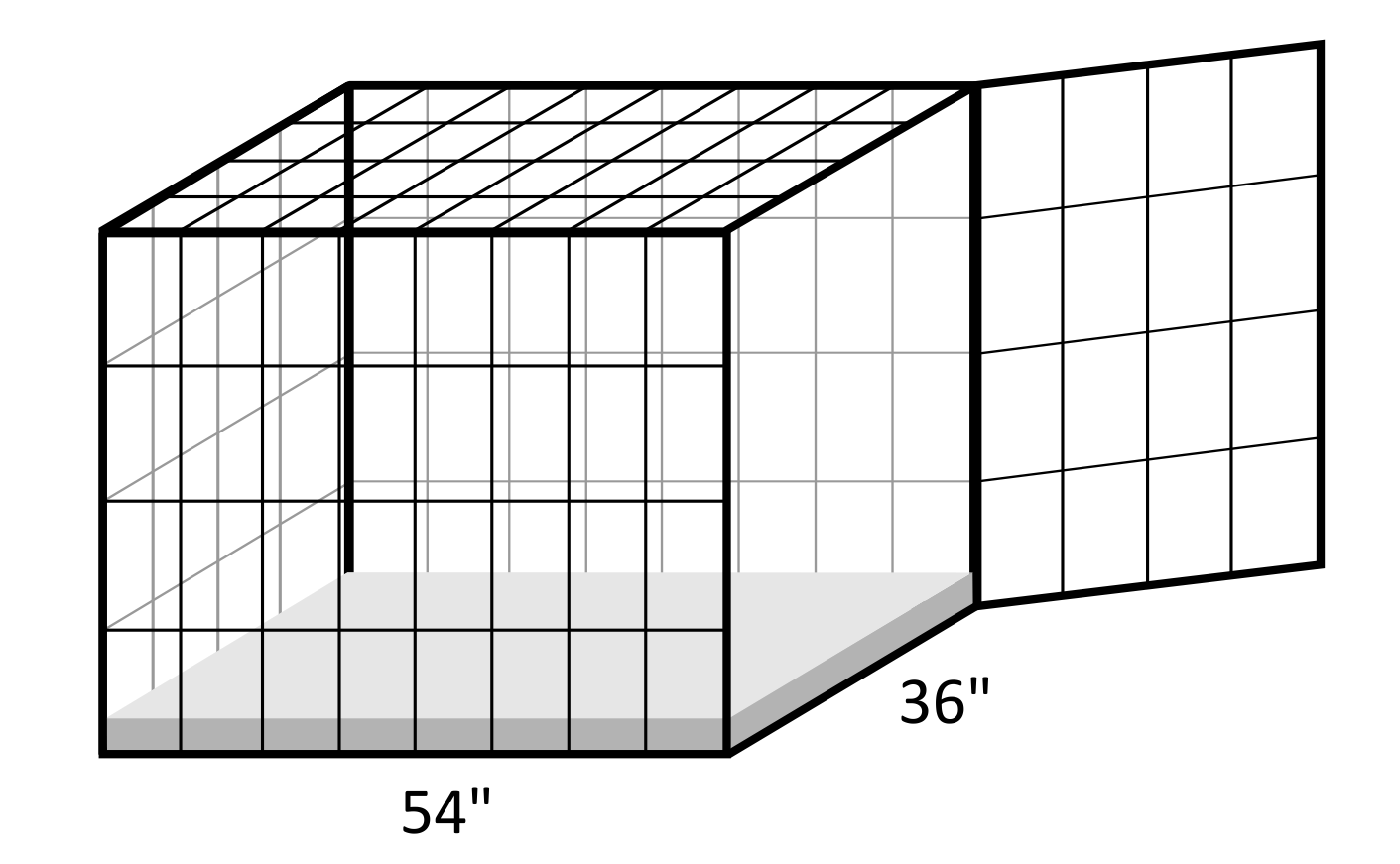# Area of a Rectangle Overview

Calculating surface area is a skill that can be applied in many real-world situations. Area calculations are made for scenarios such as determining the number of tiles needed to cover the bottom of a swimming pool, the amount of wrapping paper needed to wrap a gift, or the amount of square footage you have in your backyard. Situations such as these will require the use of surface area calculations.

Area of Rectangle Sample Questions

When determining the area of a rectangle, the formula $$A=l\times w$$ can be applied. A represents area, l represents length, and w represents width. For example, the rectangle below has a length of 32 meters and a width of 20 meters. The area of the rectangle can be calculated by multiplying $$l\times w$$, or $$32\times20$$, which is 6,400. The units associated with surface area will always be units squared. Area is the result of multiplying two dimensions, length and width, which can be represented as a power of 2. In this case, our answer would be 640 m2.Consider the following:
You want to plant grass in your new backyard, and you currently have 645 square feet of sod available. You want to determine if this is enough grass to cover the entire yard, so you need to compare the area of the sod to the area of your yard. Your yard has a length of 25 feet and a width of 30 feet. Do you have enough sod to cover the entire yard?

This is a scenario where the area formula of a rectangle can be applied. The length of the yard is 30 feet, and the width is 20 feet. The formula states that $$A=l\times w$$, so the area of the yard is $$30\text{ ft}\times25\text{ ft}$$ which is 750 square feet. In this case, 645 square feet of sod is not enough to cover the entire yard. 105 more square feet of sod will be required.

Many area problems will be presented in the format of $$A=l\times w$$, where both length and width are provided and the area is being solved for. However, it is also common in area problems to provide the area, but have missing dimensions. For example, the area of the following rectangle is 12 cm2 and its length is 6 cm. What is the width of the rectangle?The area formula can be applied in situations like this, but the formula will need to be manipulated in order to solve for w instead of A. The formula $$A=l\times w$$ becomes $$12\text{ cm}^2=6\text{ cm}\times w$$, and in order to solve for w, we need to divide both sides of the equation by 6. $$w=2\text{ cm}$$, so the width of the rectangle is 2 cm.

Consider the following:
You want to install a wire fence that surrounds your garden in order to keep deer and rabbits out. You know that the area of your garden is 210 square feet and the width is 14 feet. What is the length of the garden, and how many feet of fencing will need to be installed?

The missing length can be determined using the area formula $$A=l\times w$$. The formula becomes $$210\text{ ft}^2=l14\text{ ft}$$, and when both sides of the equation are divided by 14, the length is revealed as 15 feet. From here, we can simply add the two lengths and the two widths in order to find the total amount of fencing that is required: $$14+14+15+15=58\text{ feet of fencing}$$.

It is important to note that the formula $$A=l\times w$$ only applies to the surface area of rectangles and squares. Other two-dimensional shapes will have their own unique formulas. For example, the area of a triangle is $$A=\frac{1}{2}bh$$, and the area of a circle is $$A=\pi r^2$$.

## Area of a Rectangle Sample Questions

Here are a few sample questions going over area of a rectangle.

Question #1:

The rectangle below has a length of 4 inches and a width of 3 inches. Calculate the surface area using the area formula.15 in2

11 in2

14 in2

12 in2

The area can be calculated using the formula $$A=l\times w$$, where A represents area, l represents length, and w represents width. The formula $$A=l\times w$$ becomes $$A=4\text{ in}\times3\text{ in}$$ which simplifies to 12 in2.

Question #2:

Which scenario is an example of surface area?

The amount of water being held in a large tank

The weight of an object measured in grams

The distance around a running track in meters

The square footage of tiles covering the floor of a kitchen

Surface area can be used to describe a situation such as how many tiles are needed to completely cover the surface of a kitchen floor.

Question #3:

How many ways can you build a rectangle in order to produce a surface area of 12 square inches?

One way

Two ways

Eight ways

Six ways

The following dimensions will produce a surface area of 12 square units:
$$12\text{ in}\times1\text{ in}$$
$$1\text{ in}\times12\text{ in}$$
$$2\text{ in}\times6\text{ in}$$
$$6\text{ in}\times2\text{ in}$$
$$3\text{ in}\times4\text{ in}$$
$$4\text{ in}\times3\text{ in}$$

Question #4:

Kristen wants to insert a foam pad at the base of her dog’s sleeping crate. The crate is 54 inches in length, and 36 inches wide. What does the area of the foam pad need to be in order to fit the crate with no gaps.1,844 in2

1,744 in2

1,944 in2

1,344 in2

The surface area of the rectangular foam pad can be calculated using the area formula $$A=l\times w$$. When the dimensions are plugged into the formula, $$A=l\times w$$ becomes $$A=54\text{ in}\times36\text{ in}$$ which simplifies to 1,944 in2.

Question #5:

Andrew wants to build a pool in his backyard. His yard is 15 feet wide, so he decides to design the pool to be 10 feet wide. The surface area of the pool will be 120 square feet. How long will the pool be?

11 feet

12 feet

13 feet

14 feet

In this scenario the formula $$A=l\times w$$ can be used in order to solve for the missing side length. When the area and width are plugged into the formula, $$A=l\times w$$ becomes $$120\text{ ft}^2=l\times10\text{ ft}$$. When both sides of the equation are divided by 10, l is revealed as 12, or 12 feet for the length.